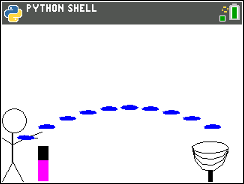# Activities

•• ##### Subject Area

• Math: Explorations with Coding: Coding with Python

6-8

• ##### Device
•TI-84 Plus CE Python

## Frisbee Golf Equations#### Activity Overview

In this coding activity for the TI-84 Plus CE Python, students will create a frisbee golf game, using Python and mathematics.

#### Objectives

• Use variables to store values
• Use the randint() function to generate random numbers.
• Use the print() function to display
• Use a while loop to repeat code.
• Solve one step equations with rational solutions. (May adjust for integers or positive numbers only.  See teacher note on step 6)
• Solve multi-step equations with rational solutions. (May skip.  See teacher note on step 20.)
• Use substitution to verify a value is a solution to an equation.